# Interpolation Algorithm for Row-Major Array Layout

This example illustrates the interpolation algorithm in 2-D and 3-D Lookup Table that is optimized for row-major array layout. The interpolation algorithm that is optimized for column-major array layout is also presented as a reference. The code generated by using row-major interpolation algorithm performs with the best speed and memory usage when operating on table data with row-major array layout. The code generated by using column-major interpolation algorithm performs best with column-major array layout.

In this example, you:

• Interpolate on a 2-D Lookup Table with column-major and row-major algorithm.

• Generate code with a row-major algorithm and an array layout.

• Identify the array layout and select the optimized algorithm.

• Interpolate on a 3-D Lookup Table with column-major and row-major algorithm.

### Simulate with 2-D Row-Major Algorithm

1. Open the example model `RowLUT2D`.

```model = 'RowLUT2D'; open_system(model); ```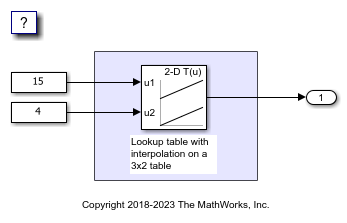2. By default, Simulink® configures a model with column-major algorithm and column-major array layout. The model `RowLUT2D` is configured to use column-major algorithm. Simulate the model. To observe the output, open the Data Inspector from the Simulation tab. The output value is 4.

3. To enable row-major algorithms, open the Configuration Parameters dialog box. On the Math and Data Types pane, enable the configuration parameter Use algorithms optimized for row-major array layout. Alternatively, in the MATLAB® Command Window, enter:

```set_param(model,'UseRowMajorAlgorithm','on'); ```

4. Simulate the model and observe those results in the Data Inspector. The output value is 4.

The column-major and row-major algorithms differ only in the interpolation order. In some cases, due to different operation order on the same data set, you might experience minor numeric differences in the outputs of column-major and row-major algorithms. For the 2-D Lookup Table used in the example model, the interpolation algorithm is illustrated here.### Generate Code by Using Row-Major Algorithm and Array Layout

The 2-D table data used in model `RowLUT2D` is:

```Table_3by2.Value ```
```ans = 1 4 2 5 3 6 ```

1. Open the Configuration Parameters dialog box. In addition to enabling the Use algorithms optimized for row-major array layout configuration parameter, on the Code Generation > Interface pane, set the configuration parameter Array layout to `Row-Major` option. This configuration parameter enables the model for row-major code generation. Alternatively, in the MATLAB Command Window, enter:

```set_param(model, 'ArrayLayout','Row-major'); ```

2. Change your current folder in MATLAB to a writable folder. On the C Code tab, click Build to generate C code.

3. In the generated code, observe the table data with row-major array layout. For comparison, here is the table data in the generated code for column-major array layout.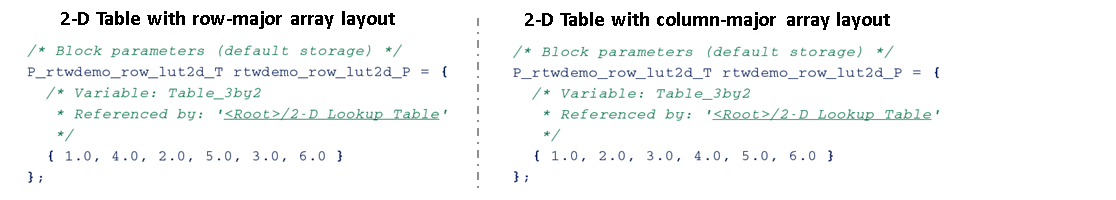In the generated code, the table data is in row-major order and the interpolation algorithm is optimized for row-major array layout. The row-major algorithm operates on table data that is contiguous in memory. This leads to faster cache access, making these algorithms cache-friendly.

This table summarizes the relationship between array layout and cache-friendly algorithms. It is recommended to use the algorithm that is optimized for the specified array layout to achieve good performance. For example, use row-major interpolation algorithm when the array layout is set to `Row-Major` for code generation.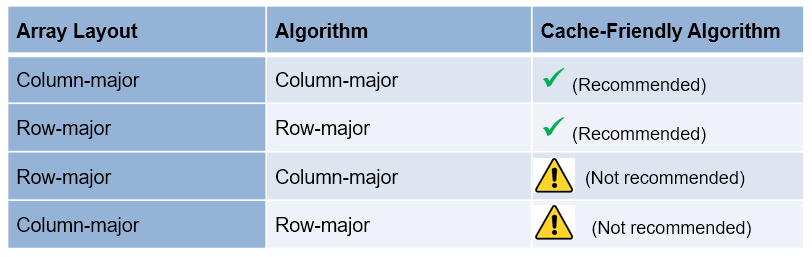### Interpolation on a 3-D Table

1. Open the example model `RowInterpolationAlgorithm`.

```open_system('RowInterpolationAlgorithm'); ```2. Generate code for the model by using the preceding procedure. Simulate and generate code from the model by repeating the steps performed on `RowLUT2D` model.

The row-major and column-major interpolations on the 3-D table used in the example model are illustrated here.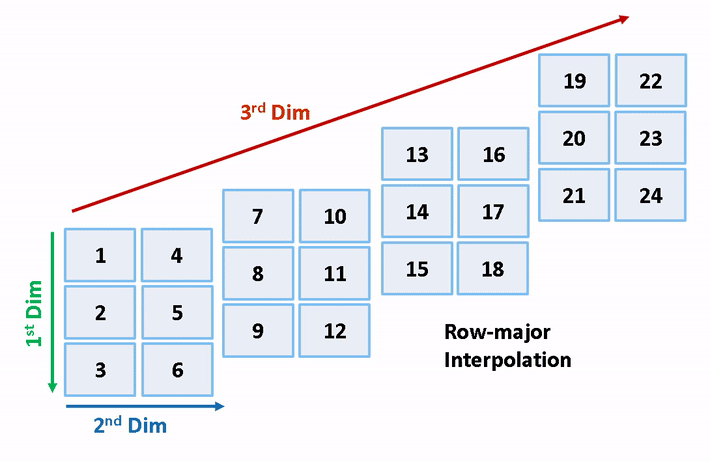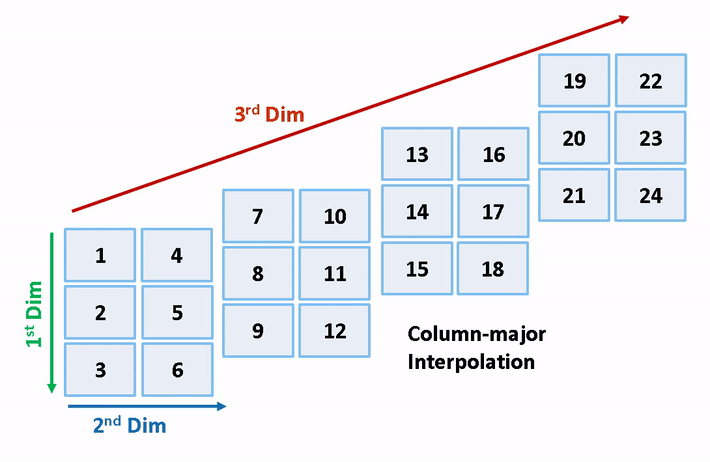```close_system('RowLUT2D',0); close_system('RowInterpolationAlgorithm', 0); ```